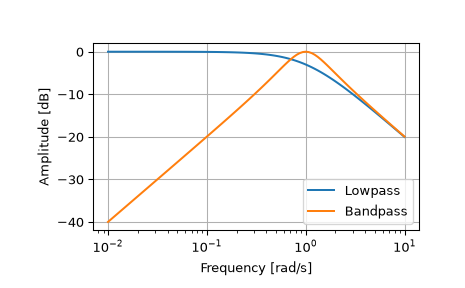# scipy.signal.lp2bp¶

scipy.signal.lp2bp(b, a, wo=1.0, bw=1.0)[source]

Transform a lowpass filter prototype to a bandpass filter.

Return an analog band-pass filter with center frequency wo and bandwidth bw from an analog low-pass filter prototype with unity cutoff frequency, in transfer function (‘ba’) representation.

Parameters
barray_like

Numerator polynomial coefficients.

aarray_like

Denominator polynomial coefficients.

wofloat

Desired passband center, as angular frequency (e.g., rad/s). Defaults to no change.

bwfloat

Desired passband width, as angular frequency (e.g., rad/s). Defaults to 1.

Returns
barray_like

Numerator polynomial coefficients of the transformed band-pass filter.

aarray_like

Denominator polynomial coefficients of the transformed band-pass filter.

Notes

This is derived from the s-plane substitution

$s \rightarrow \frac{s^2 + {\omega_0}^2}{s \cdot \mathrm{BW}}$

This is the “wideband” transformation, producing a passband with geometric (log frequency) symmetry about wo.

Examples

>>> from scipy import signal
>>> import matplotlib.pyplot as plt

>>> lp = signal.lti([1.0], [1.0, 1.0])
>>> bp = signal.lti(*signal.lp2bp(lp.num, lp.den))
>>> w, mag_lp, p_lp = lp.bode()
>>> w, mag_bp, p_bp = bp.bode(w)

>>> plt.plot(w, mag_lp, label='Lowpass')
>>> plt.plot(w, mag_bp, label='Bandpass')
>>> plt.semilogx()
>>> plt.grid()